# Conic sections questions and answers

Recent questions in Conic sectionsusagirl007A 2021-09-18 Answered

### At one point in a pipeline the water’s speed is 3.00 m/s and the gauge pressure is $$\displaystyle{5.00}\times{10}^{{{4}}}{P}{a}$$. Find the gauge pressure at a second point in the line, 11.0 m lower than the first, if the pipe diameter at the second point is twice that at the first.Rivka Thorpe 2021-08-14 Answered

### A conic section is said to be circle if the eccentricity e=1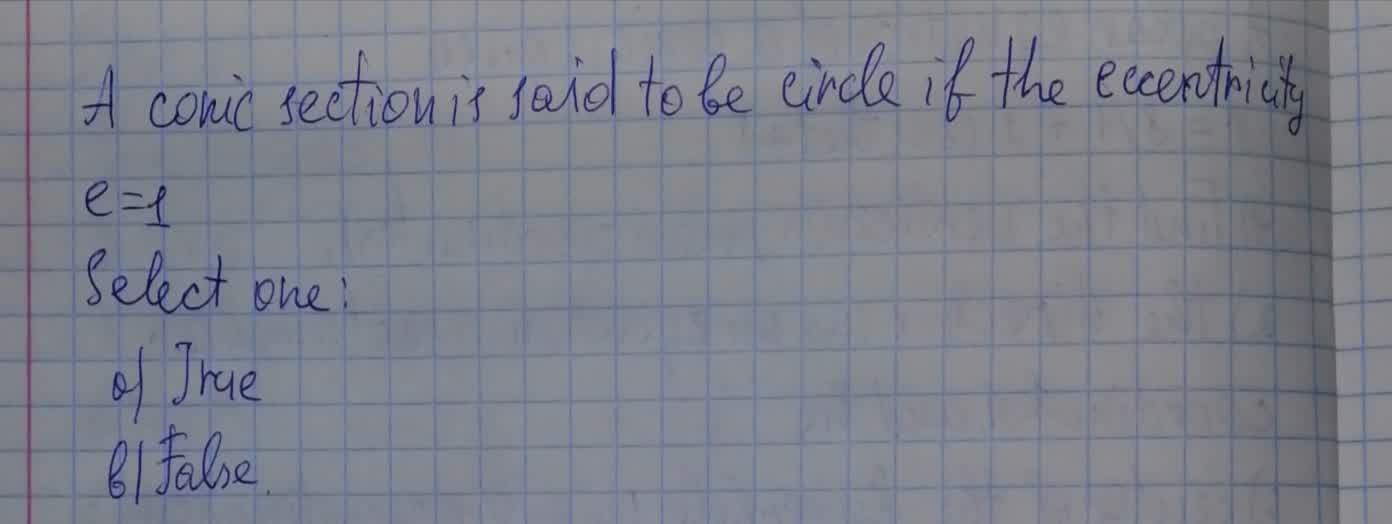kuCAu 2021-08-14 Answered

### Identify and graph the conic whose equation is in attachment. $$\displaystyle{5}{x}^{{2}}+{4}{x}{y}+{2}{y}^{{2}}-{\frac{{{28}}}{{\sqrt{{5}}}}}{x}-{\frac{{{4}}}{{\sqrt{{5}}}}}{y}+{4}={0}$$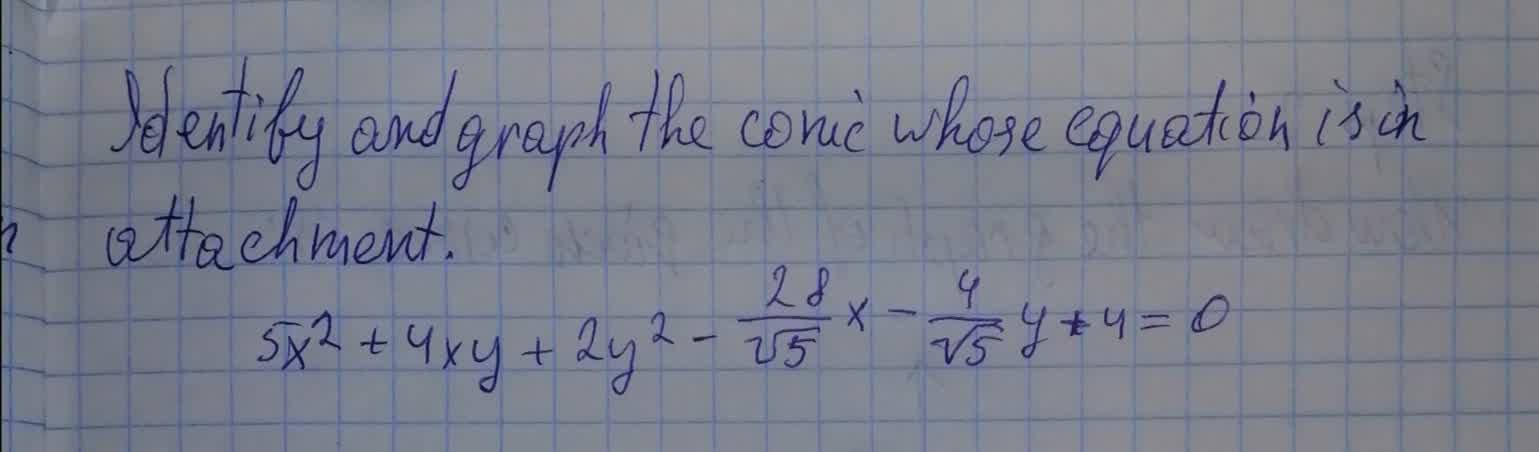Bevan Mcdonald 2021-08-14 Answered

### Identify each conic using eccentricity. (a) $$r=\frac{4}{1+3\sin \theta}$$ (b) $$r=\frac{7}{1-3\cos \theta}$$ (c) $$r=\frac{8}{6+5\cos \theta}$$ (d) $$r=\frac{3}{2-3\sin \theta}$$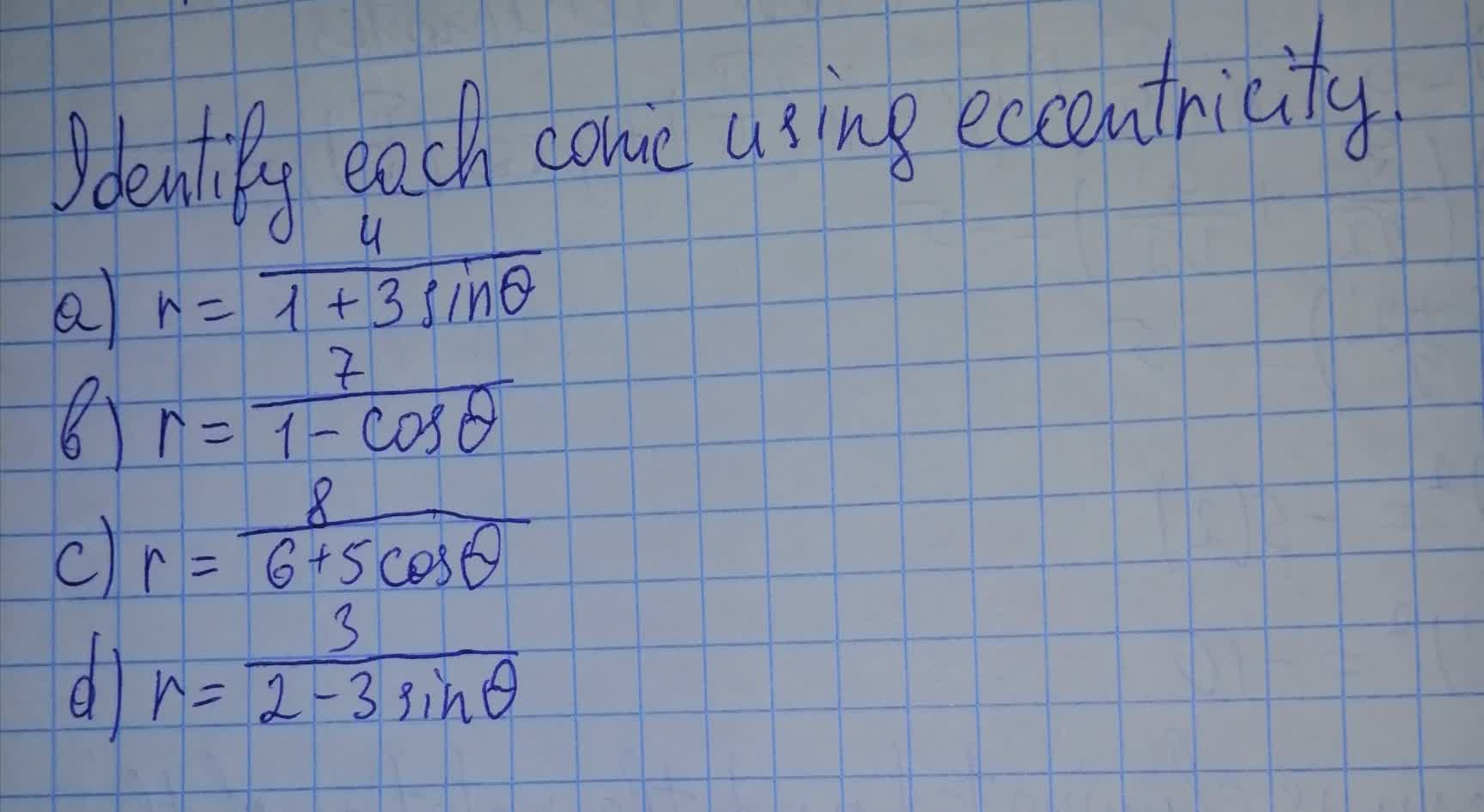nicekikah 2021-08-13 Answered

### The front (and back) of a greenhouse have the same shape and dimensions shown below. The greenhouse is 40 feet long and the angle at the top of the roof is $$\displaystyle{90}^{{\circ}}$$. Determine the volume of the greenhouse in cubic feet. Explain your solution.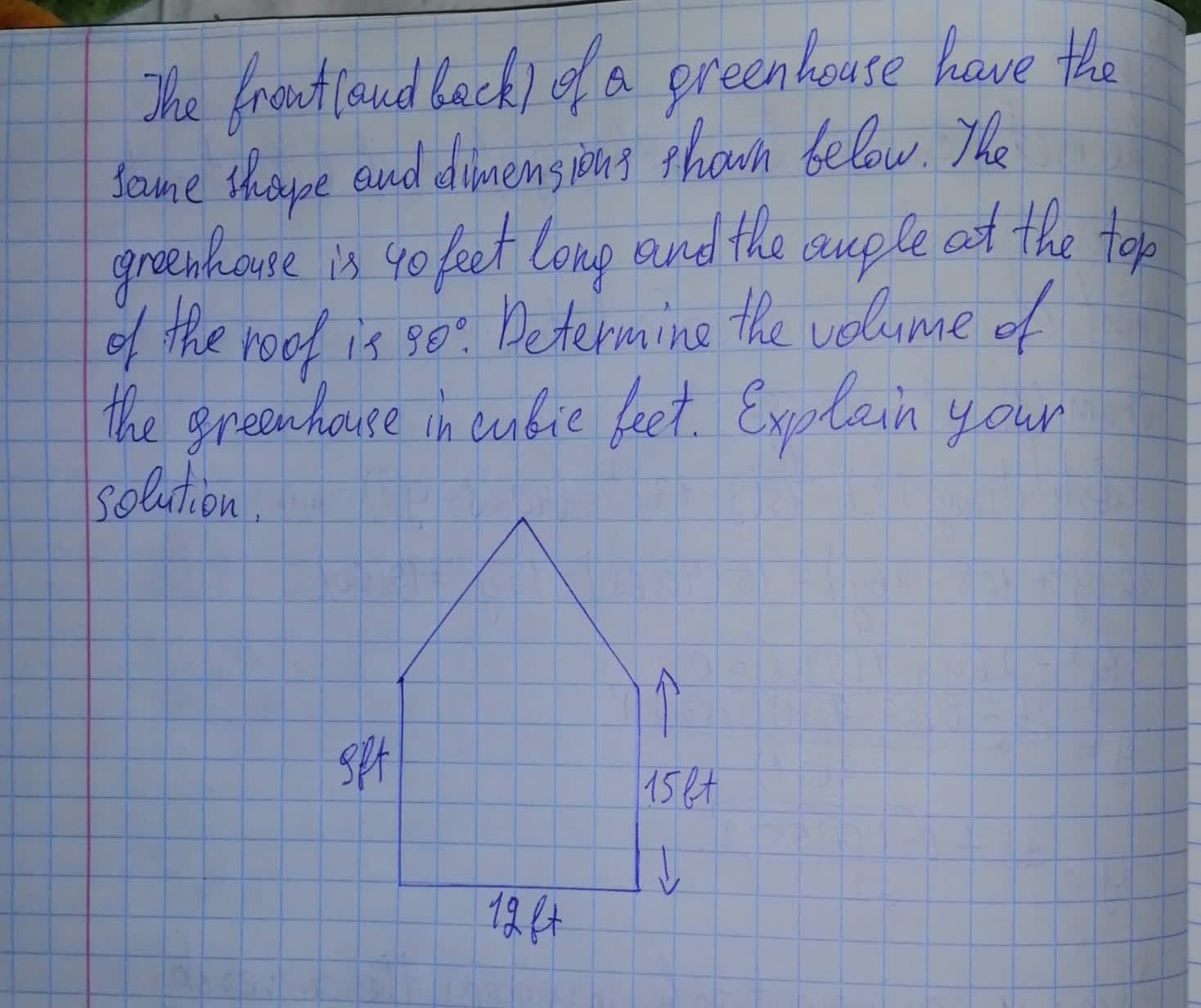Armorikam 2021-08-13 Answered

### Your mission is to track incoming meteors to predict whether or not they will strike Earth. Since Earth has a circular cross section, you decide to set up a coordinate system with its origin at Earth's center. The equation of Earth's surface is $$\displaystyle{x}^{{2}}+{y}^{{2}}={40.68}$$, where x and y are distances in thousands of kilometers.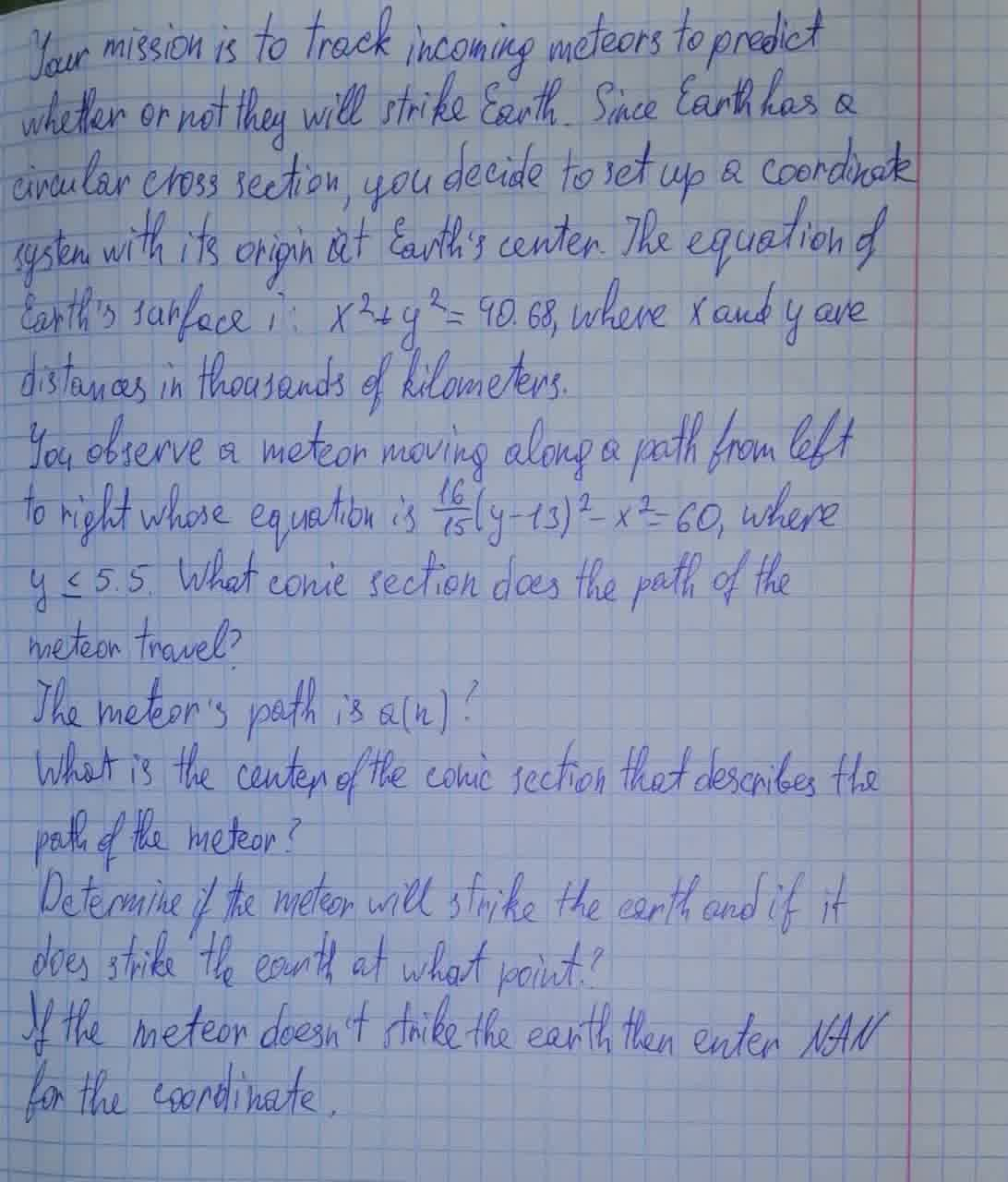nicekikah 2021-08-13 Answered

### Find an equation of the conic described.Graph the equation. Ellipse; center at (0,0); focus at (0,3); vertex at (0, 5)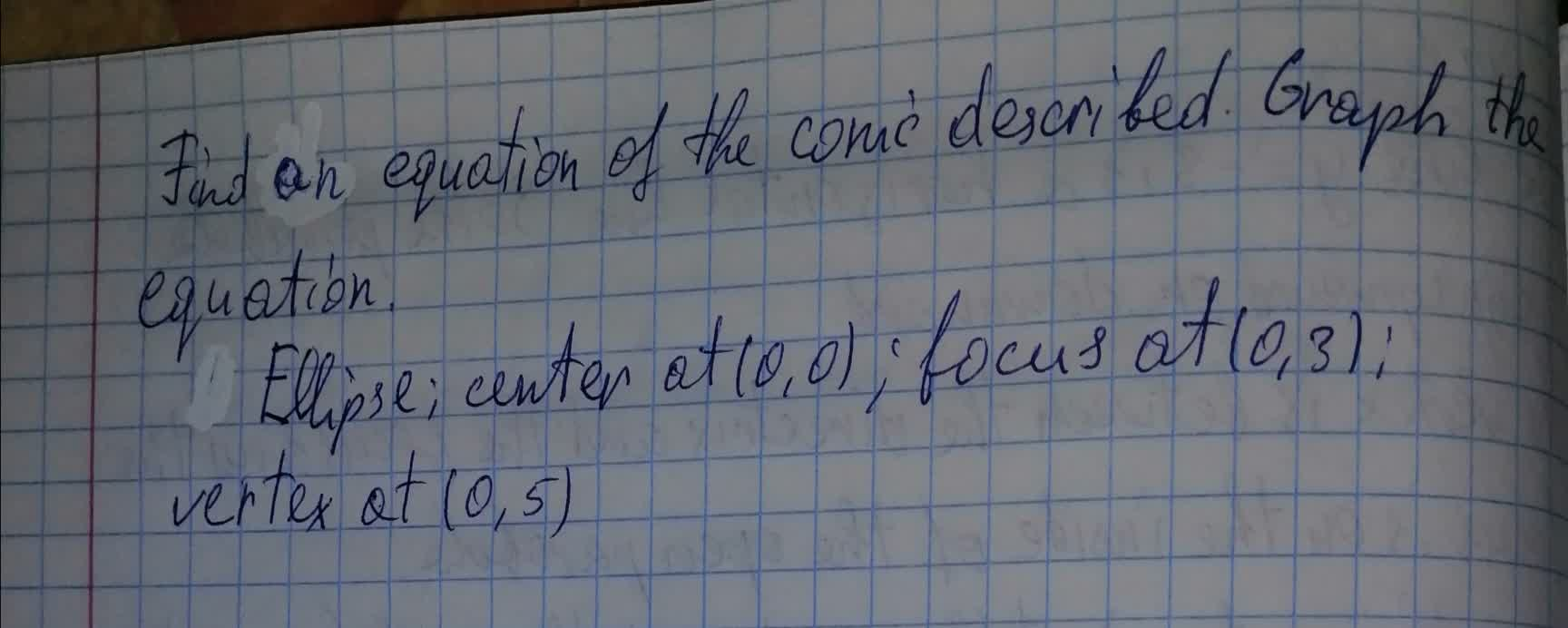Anonym 2021-08-12 Answered

### To determine: Find the lateral area of the conical tent.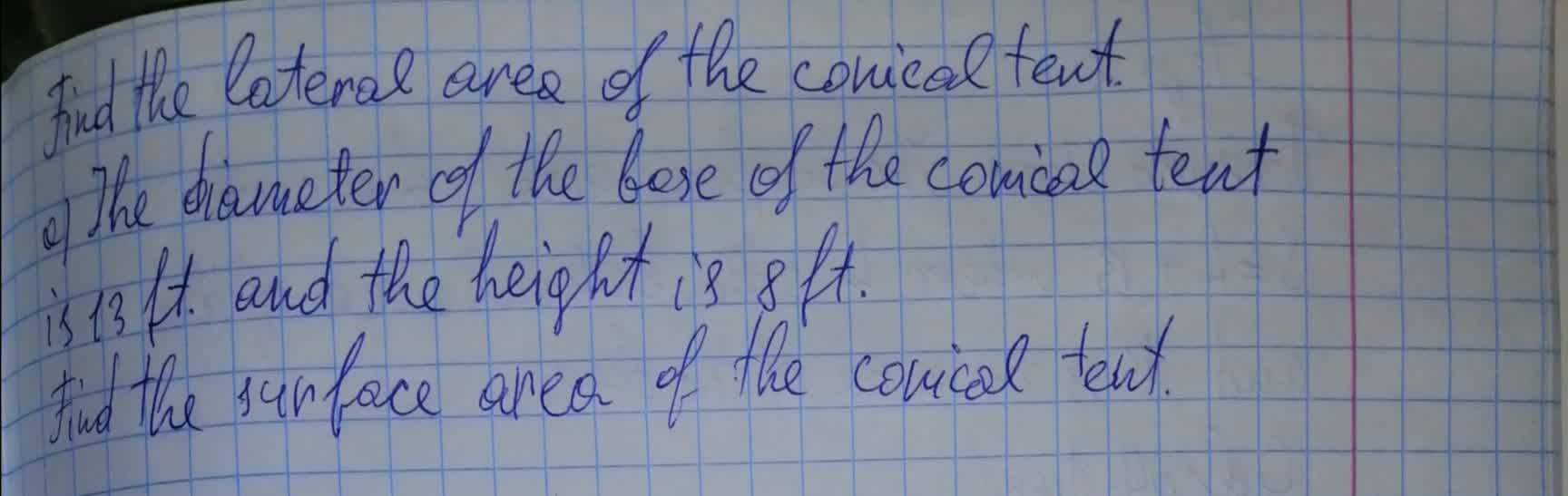Emeli Hagan 2021-08-12 Answered

### Find the focus, equation of the directrices, lengths of major axis, minor axis and focal diameters, and draw the conic defined by $$\displaystyle{9}{x}^{{2}}+{16}{y}^{{2}}={1}$$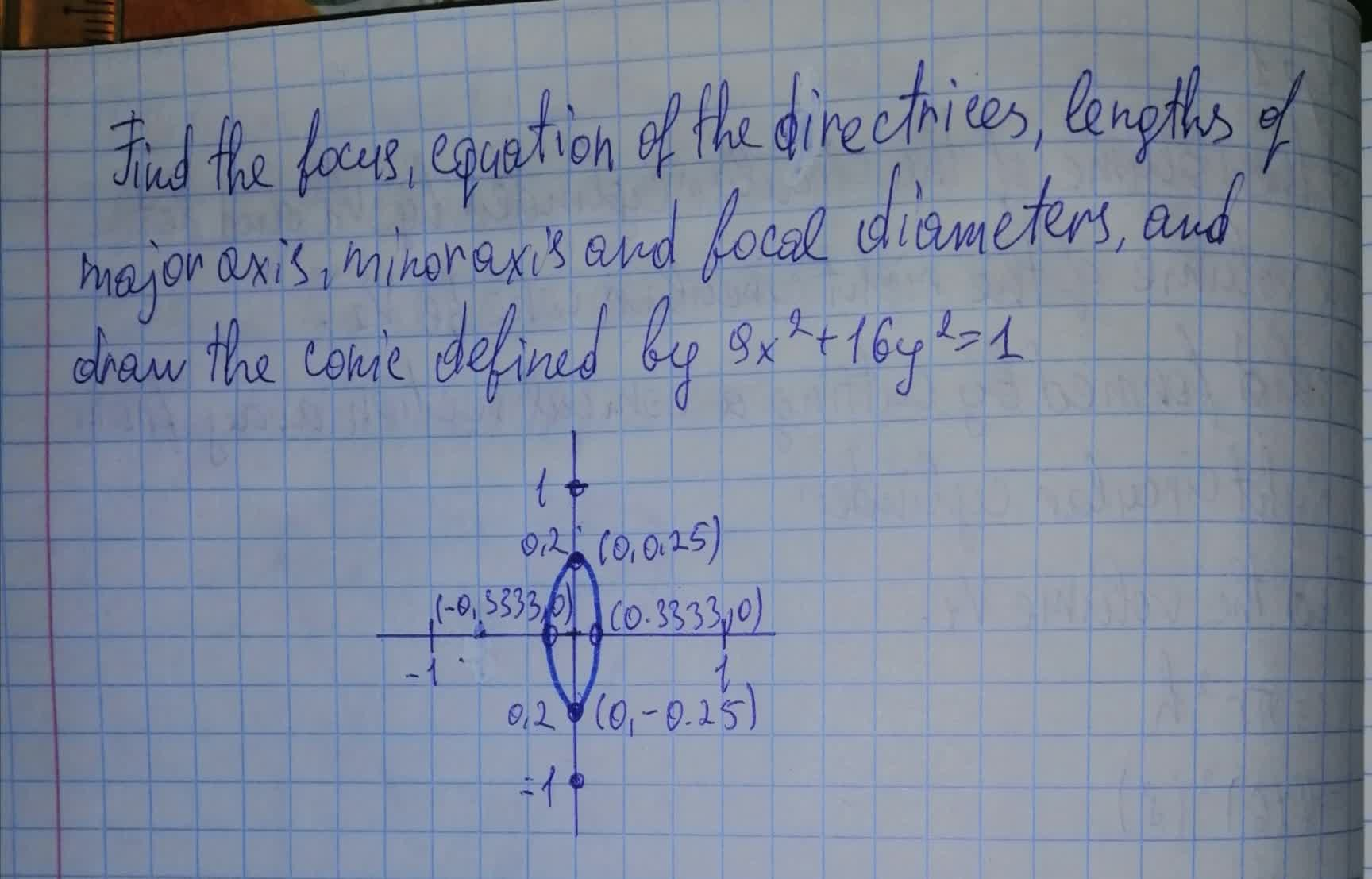Ernstfalld 2021-08-12 Answered

### Identify what type of conic section is given by the equation below and then find the center, foci, and vertices. If it is a hyperbola, you should also find the asymptotes. $$\displaystyle{4}{x}^{{2}}-{24}{x}-{4}{y}+{28}={y}^{{2}}$$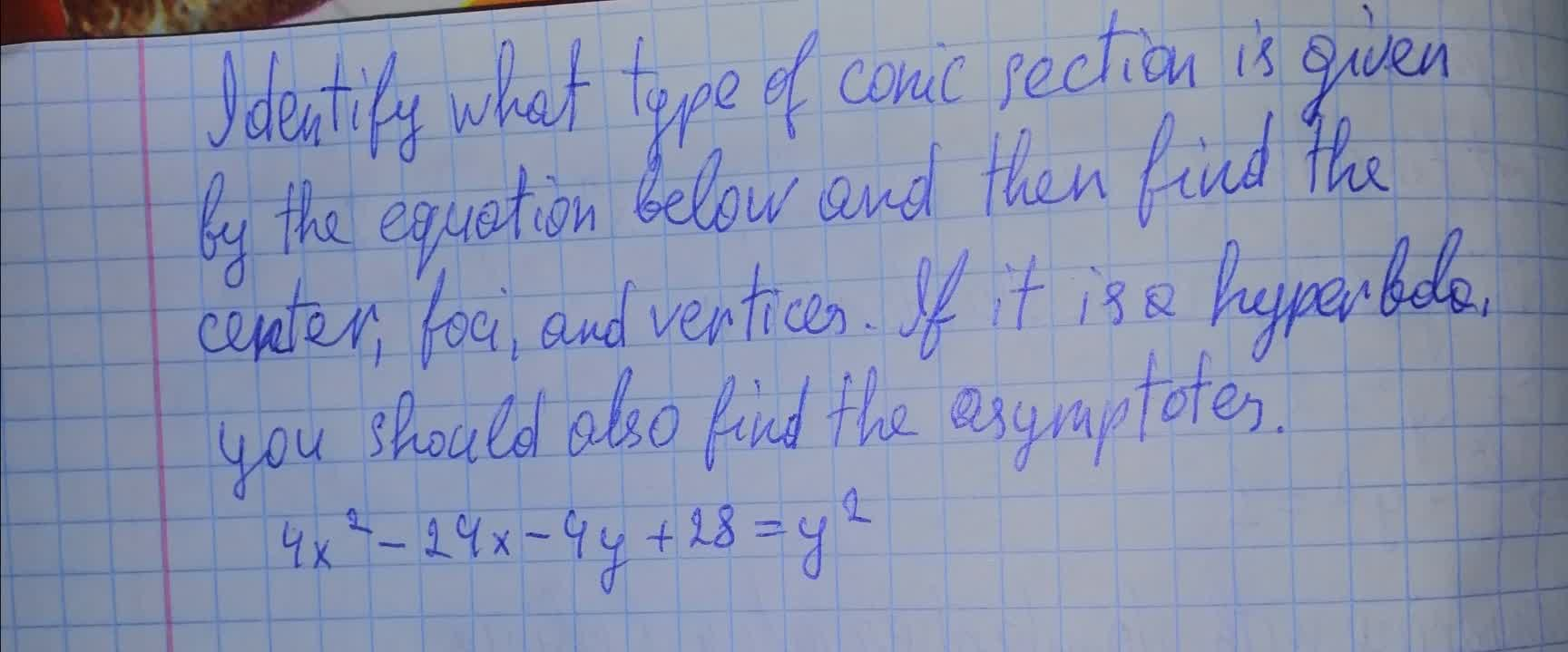chillywilly12a 2021-08-12 Answered

### Find the focus, equation of the directrices, lengths of major axis, minor axis and focal diameters, and draw the conic defined by $$\displaystyle{4}{x}^{{2}}+{7}{y}^{{2}}={1}$$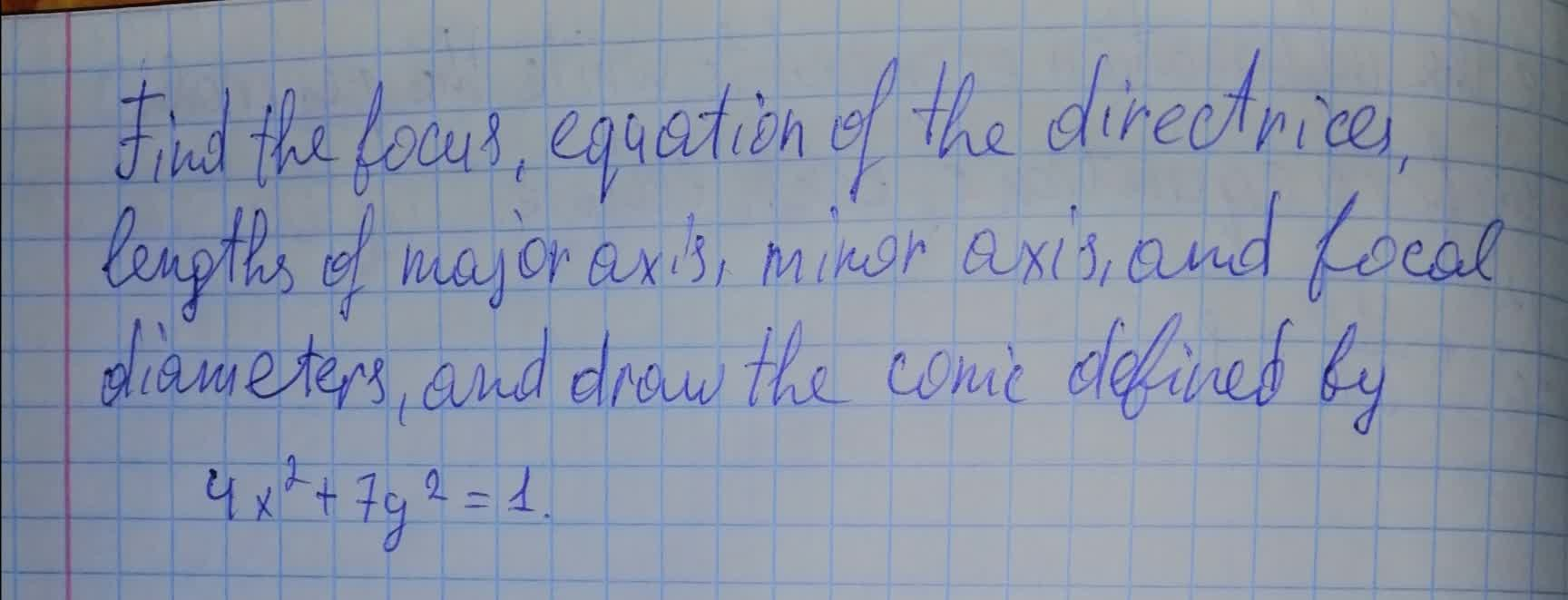abondantQ 2021-08-12 Answered

### Solve for the equation in standard form of the following conic sections and graph the curve on a Cartesian plane indicating important points (i.e. vertices and intercepts).The hyperbola given by $$\displaystyle{5}{x}^{{2}}–{4}{y}^{{2}}={20}{x}+{24}{y}+{36}$$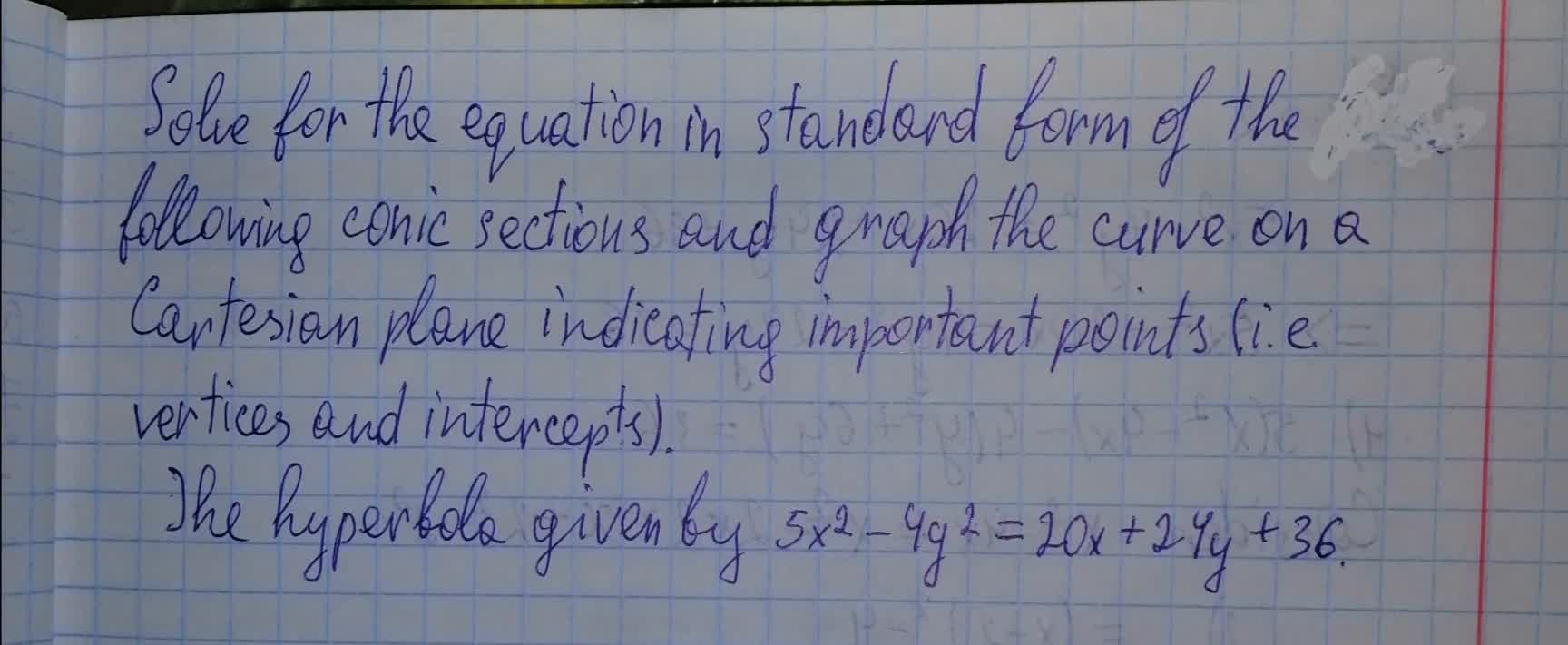Tahmid Knox 2021-08-12 Answered

### Show that for eccentricity equal to one in Equation 13.10 for conic sections, the path is a parabola. Do this by substituting Cartesian coordinates, x and y, for the polar coordinates, r and θ , and showing that it has the general form for a parabola, $$\displaystyle{x}={a}{y}^{{2}}+{b}{y}+{c}$$ .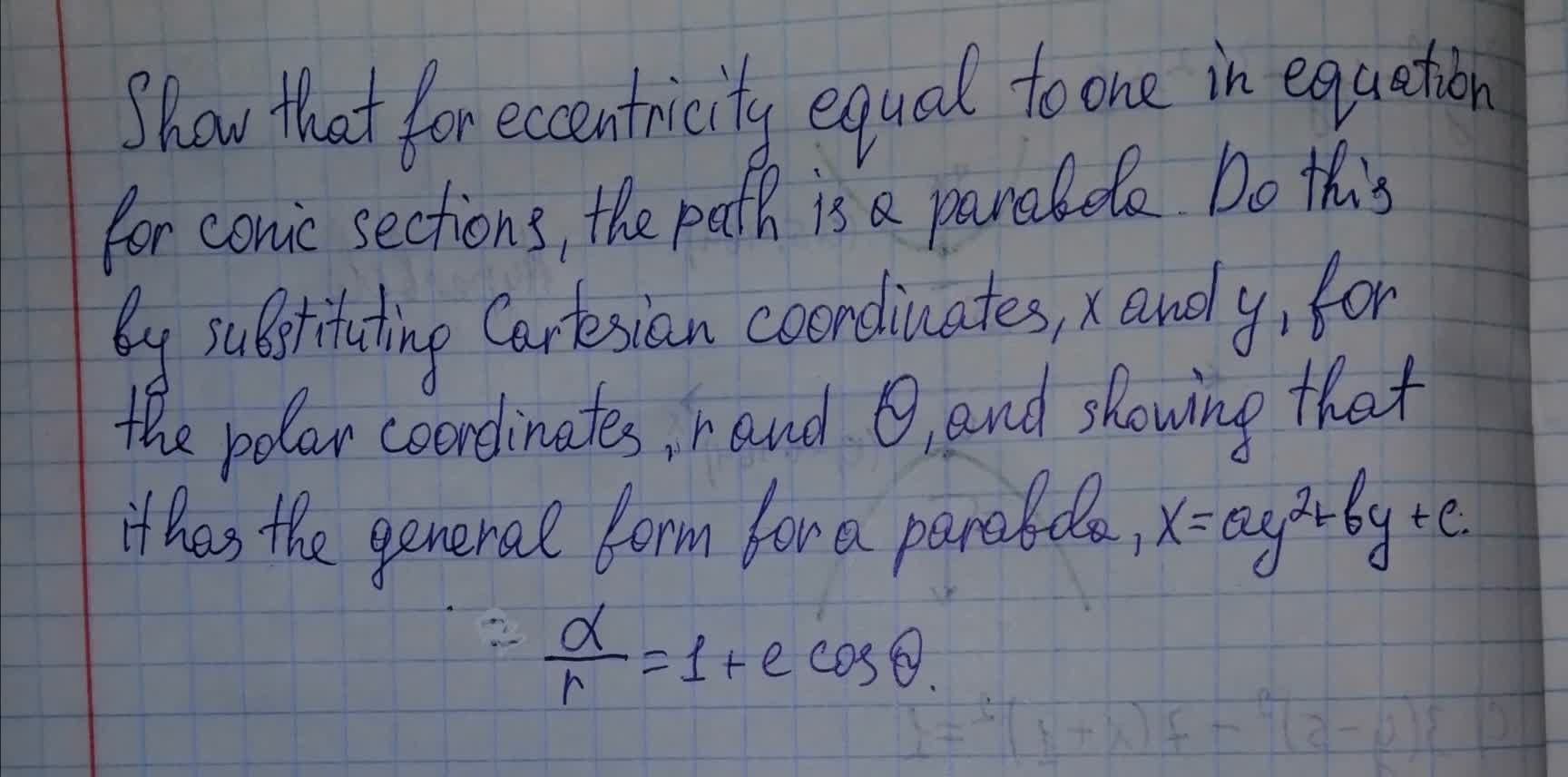Tazmin Horton 2021-08-11 Answered

### $$\displaystyle{S}_{{1}},{S}_{{2}}$$ are two foci of the ellipse $$\displaystyle{x}^{{2}}+{2}{y}^{{2}}={2}$$. P be any point on the ellipse The locus incentre of the triangle $$\displaystyle{P}{S}{S}_{{1}}$$, is a conic where length of its latus rectum is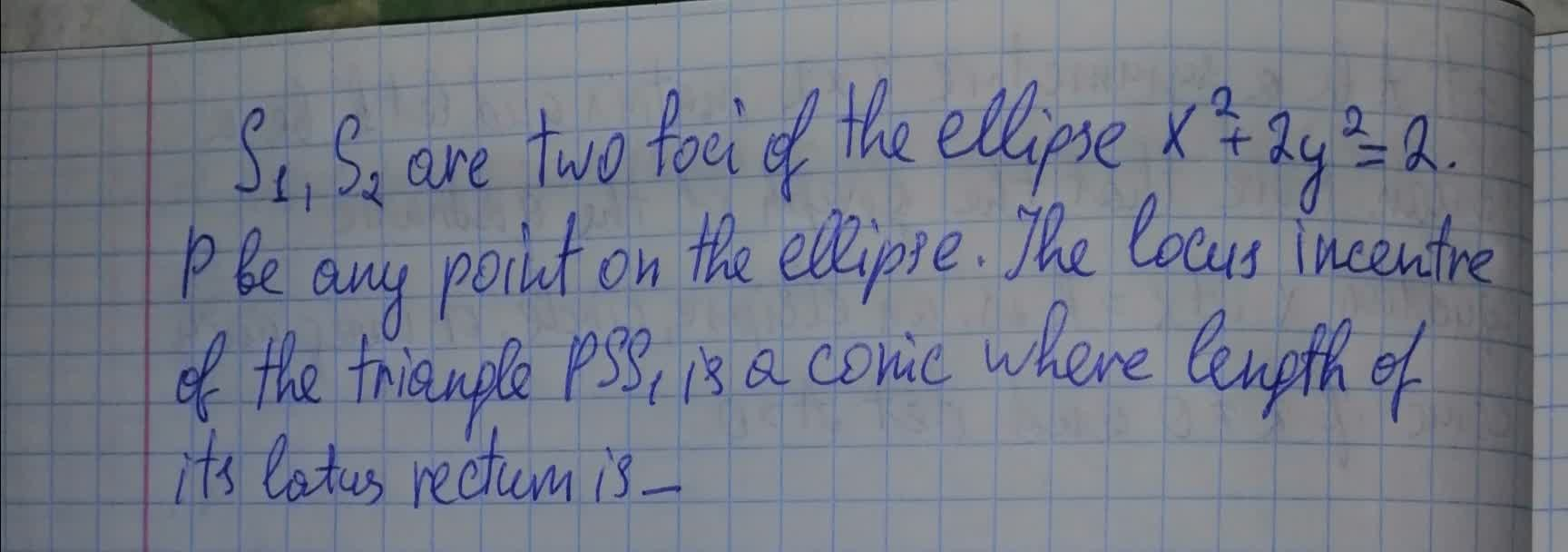tabita57i 2021-08-11 Answered

### Find the vertices and foci of the conic section. $$\frac{x^2}{4 }− \frac{y^2}{9} = 36$$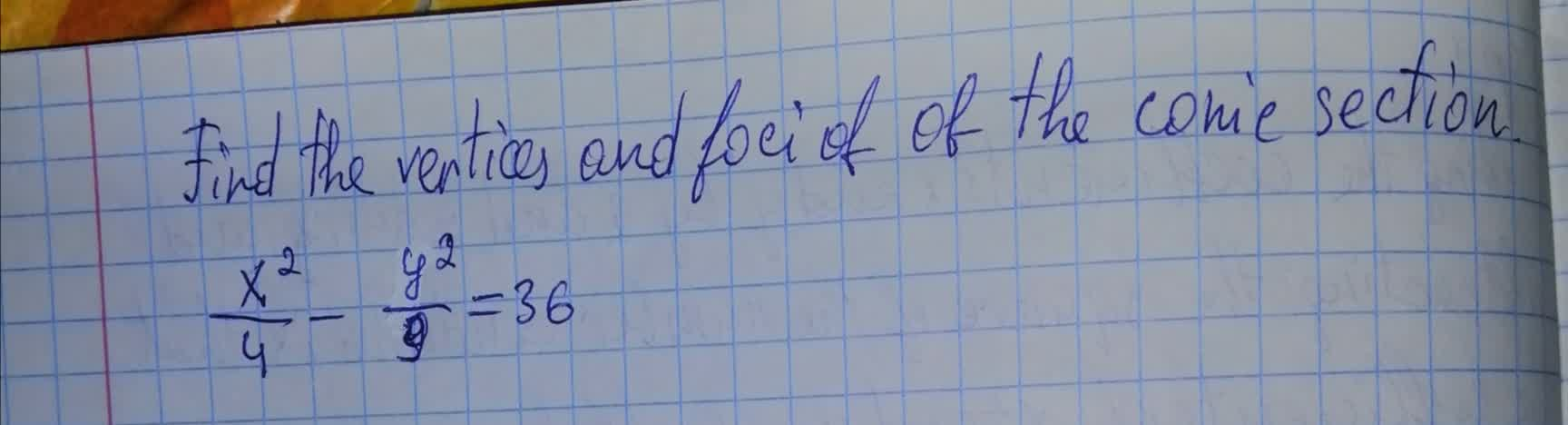Wierzycaz 2021-08-11 Answered

### Find the equation of the graph for each conic in standard form. Identify the conic, the center, the vertex, the co-vertex, the focus (foci), major axis, minor axis, $$\displaystyle{a}^{{2}},{b}^{{2}}$$, and $$\displaystyle{c}^{{2}}$$?. For hyperbola, find the asymptotes. Sketch the graph. $$\displaystyle{9}{x}^{{2}}+{25}{y}^{{2}}+{54}{x}-{50}{y}-{119}={0}$$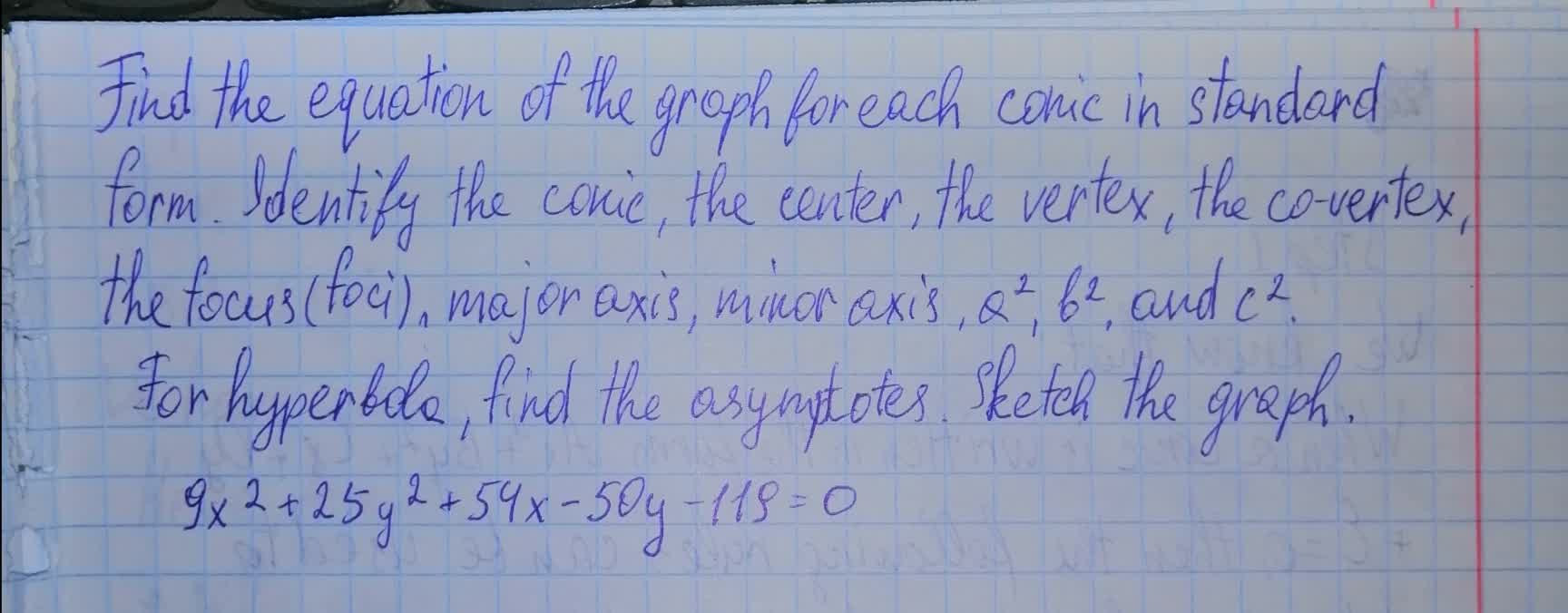Tolnaio 2021-08-11 Answered

### Find the equation of the graph for each conic in general form. Identify the conic, the center, the vertex, the co-vertex, the focus (foci), major axis, minor axis, $$\displaystyle{a}^{{2}}$$, $$\displaystyle{b}^{{2}}$$, and $$\displaystyle{c}^{{2}}$$. For hyperbola,find the asymtotes.Sketch the graph $$\displaystyle{16}{x}^{{2}}-{4}{y}^{{2}}-{64}={0}$$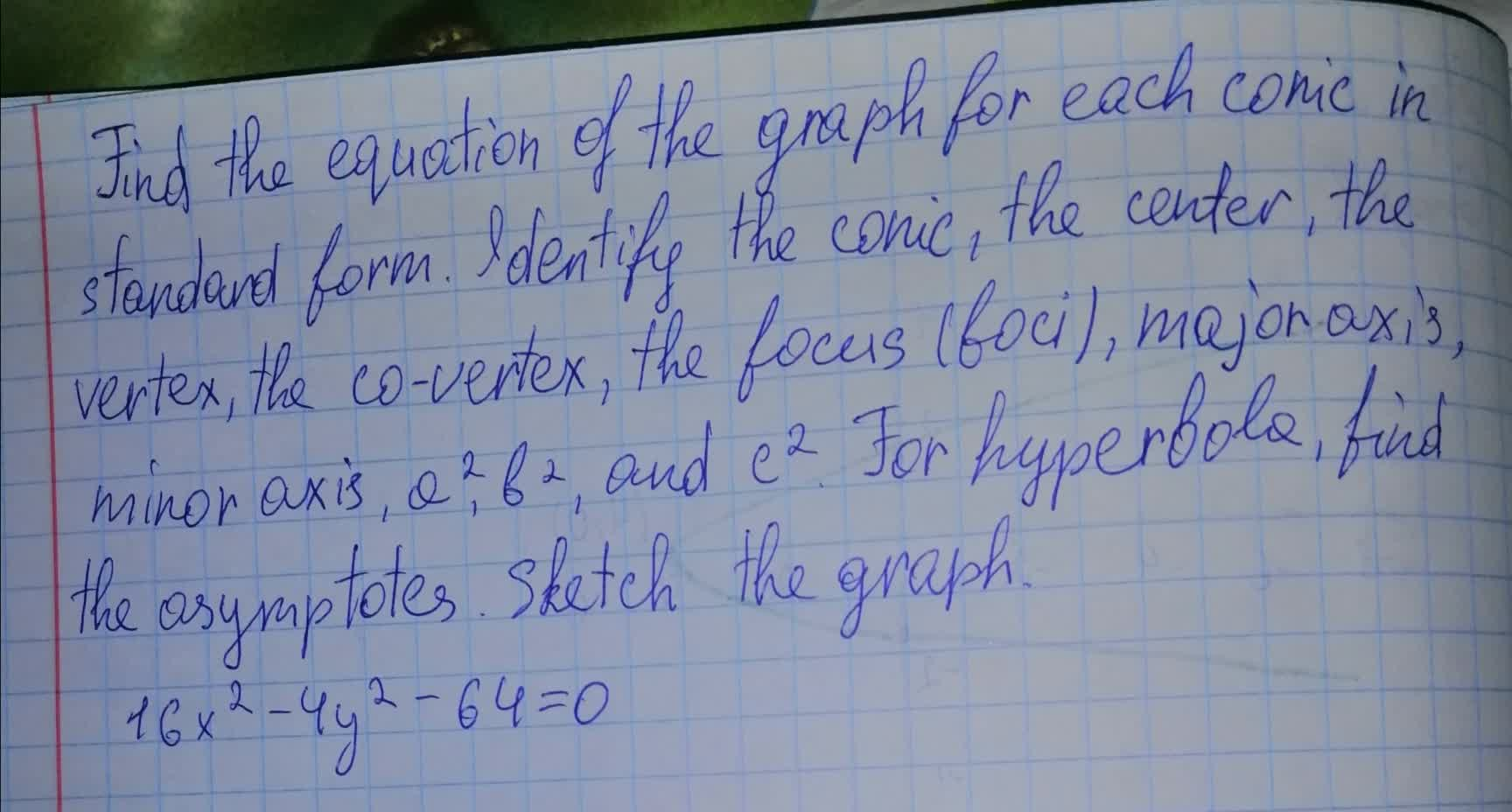pancha3 2021-08-10 Answered

### Show that the graph of an equation of the form $$\displaystyle{A}{x}^{{2}}+{C}{y}^{{2}}+{D}{x}+{E}{y}+{F}={0},{A}\ne{0},{C}\ne{0}$$ where A and C are of opposite sign, (a) is a hyperbola if $$\displaystyle{\frac{{{D}^{{2}}}}{{{4}{A}}}}+{\frac{{{E}^{{2}}}}{{{4}{C}}}}-{F}\ne{0}$$ (b) is two intersecting lines if $$\displaystyle{\frac{{{D}^{{2}}}}{{{4}{A}}}}+{\frac{{{E}^{{2}}}}{{{4}{C}}}}-{F}={0}$$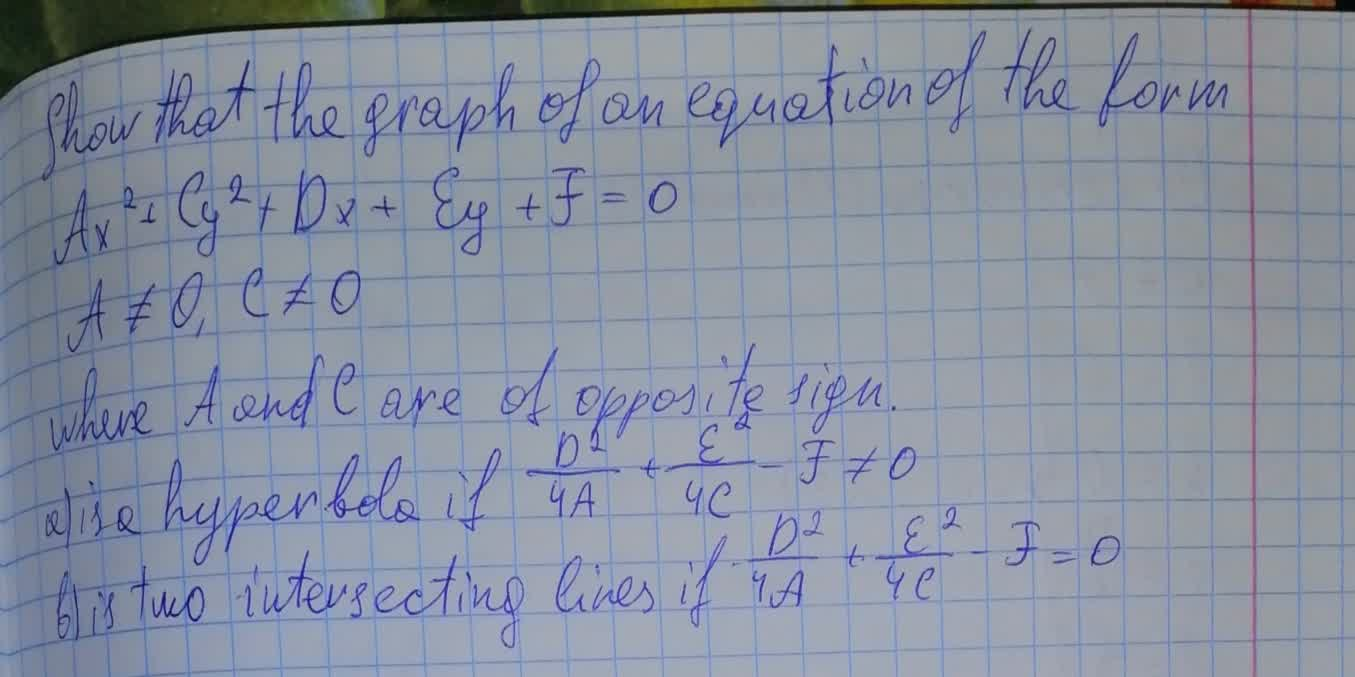nitraiddQ 2021-08-10 Answered

### Let A be a symmetric $$\displaystyle{2}\times{2}$$ matrix and let k be a scalar. Prove that the graph of the quadratic equation $$\displaystyle{x}^{{T}}$$ Ax=k is ,an ellipse, circle, or imaginary conic if $$\displaystyle{k}\ne{q}{0}$$ and det A > 0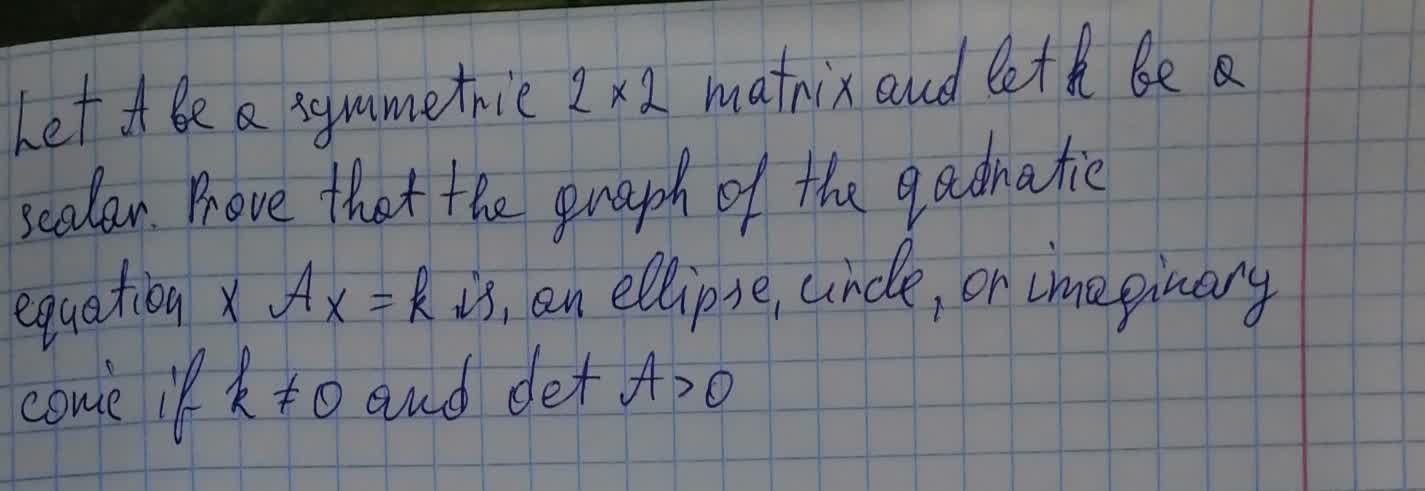Sinead Mcgee 2021-08-10 Answered

...